RS Aggarwal Class 8 Solutions Chapter 1 - Rational Numbers

RS Aggarwal Class 8 Chapter 1 - Rational Numbers Solutions Free PDF

In this chapter, the students will learn about the rational number and its properties. Like closure property, the property of commutativity, the property of associativity. In the second part, the students will learn how to find a rational number between two rational numbers. Solve the questions from the RS Aggarwal class 8 to learn the concepts of the rational number is an easy way. For a better understanding of RS Aggarwal questions, students can refer to RS Aggarwal class 8 solutions chapter 1 rational numbers.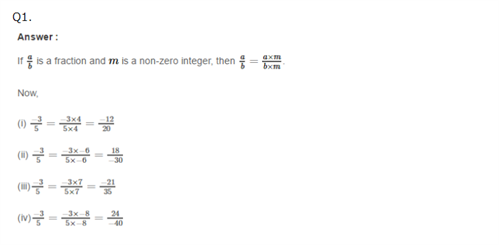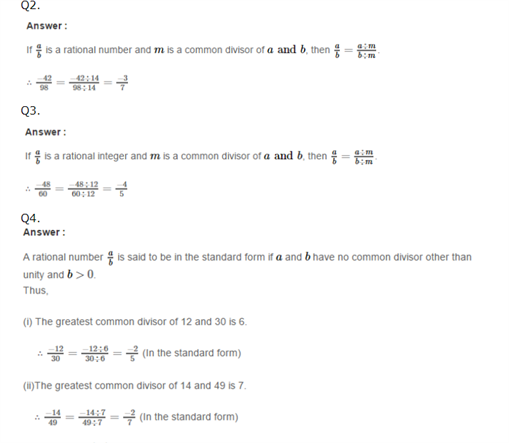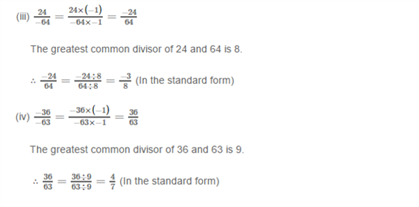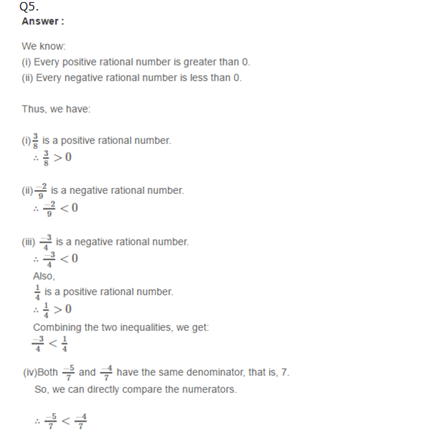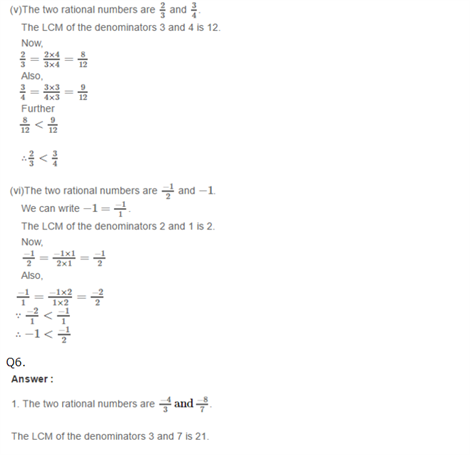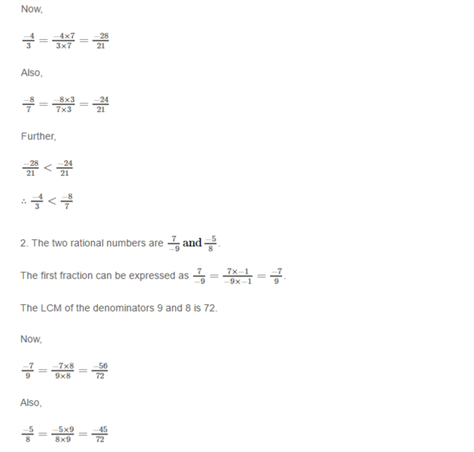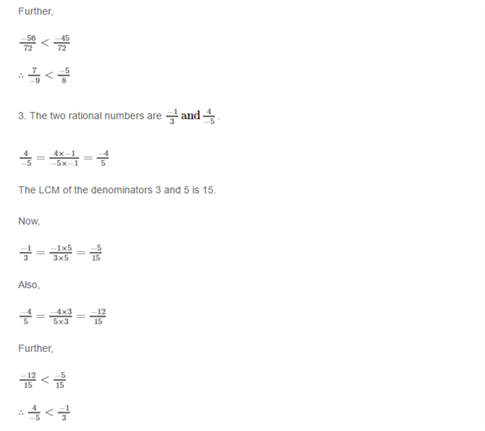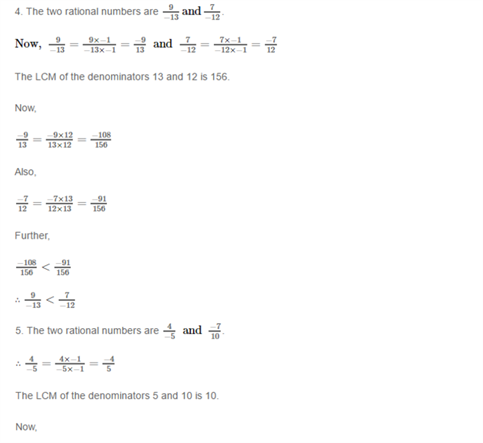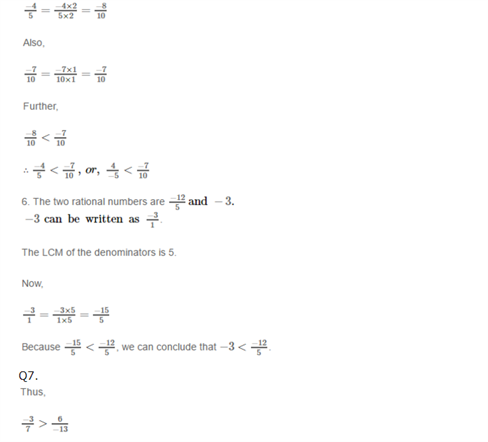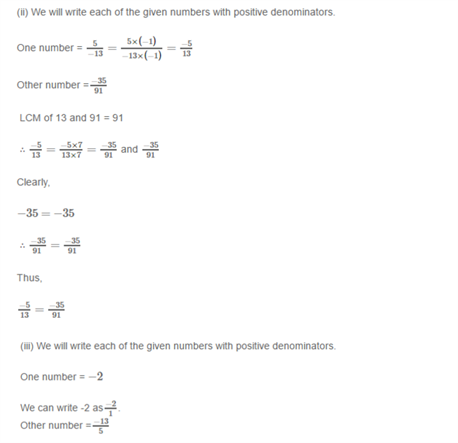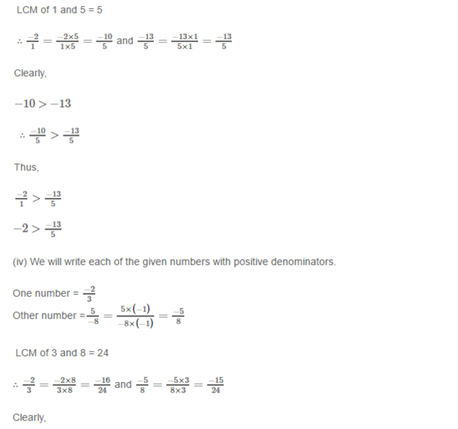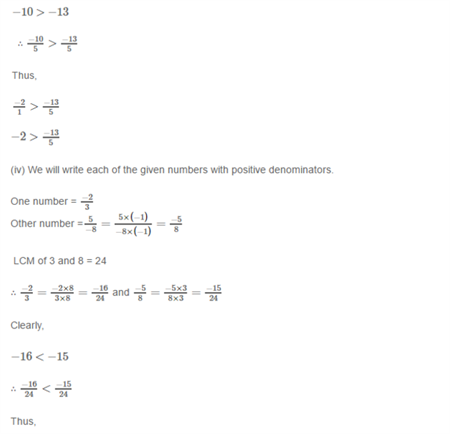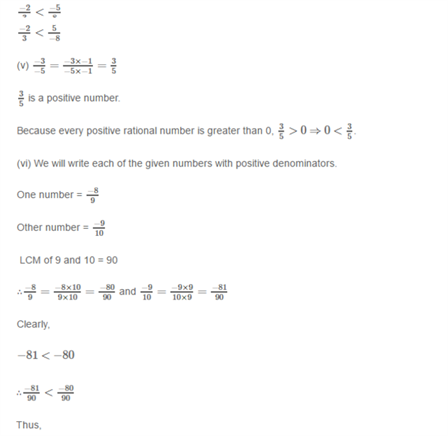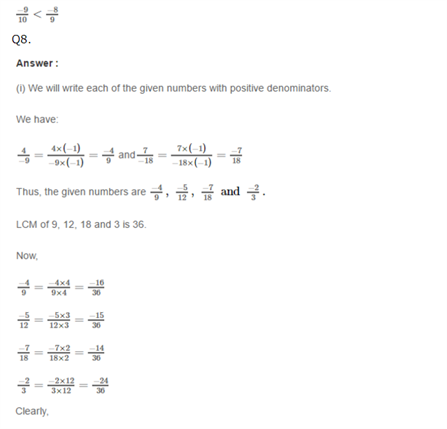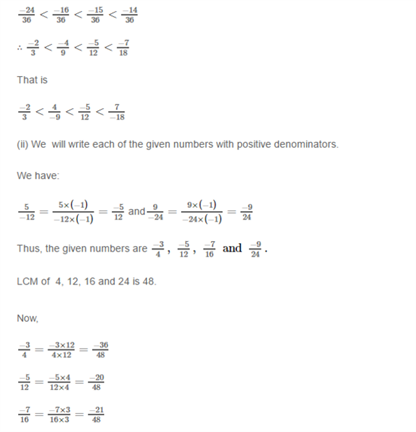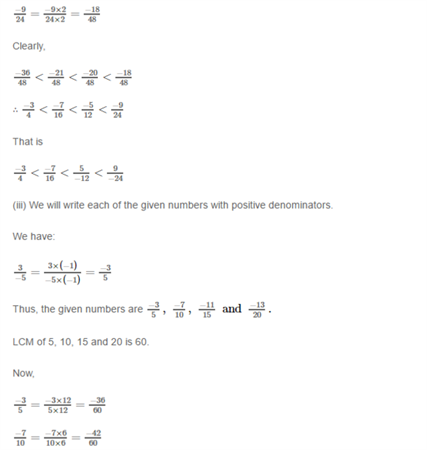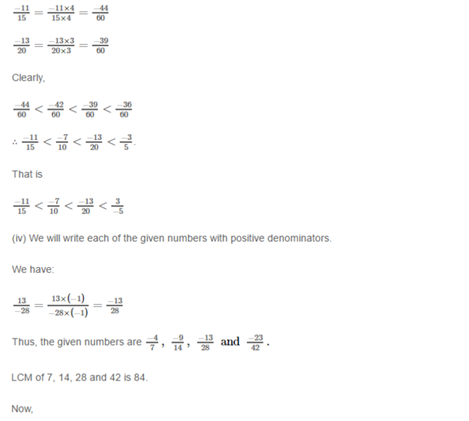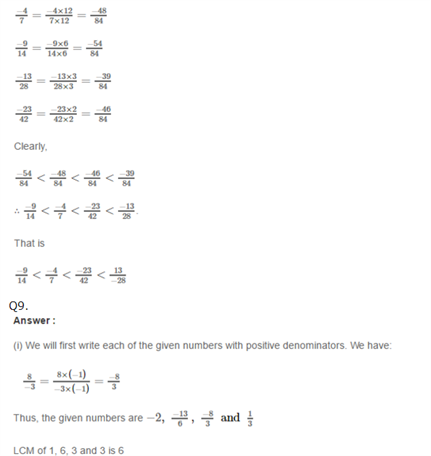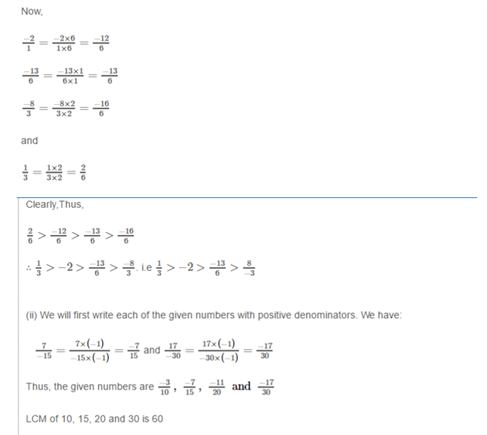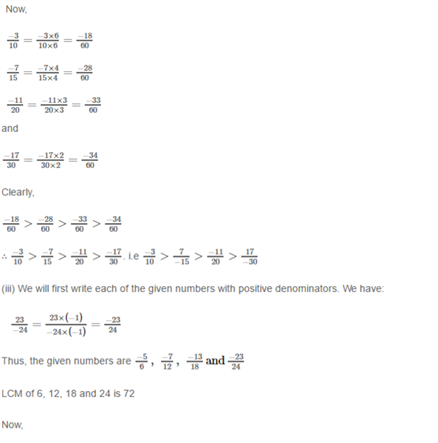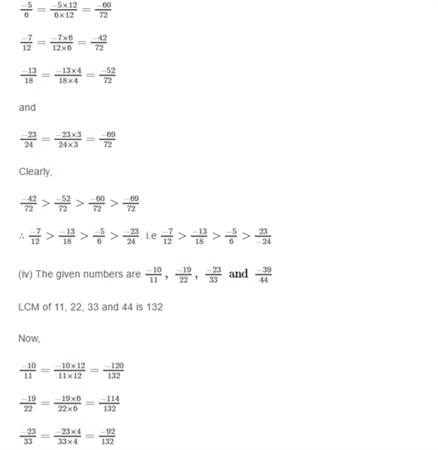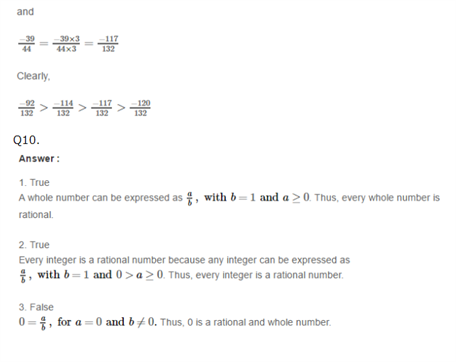Practise This Question

In the construction of quadrilateral PQRS, with PQ = 3 cm, QR = 4 cm and PS = 5 cm, also, SPQ = 70 and PQR = 90, which of the sides is to be taken as base and constructed first?